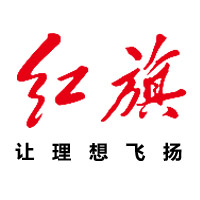# 红旗H7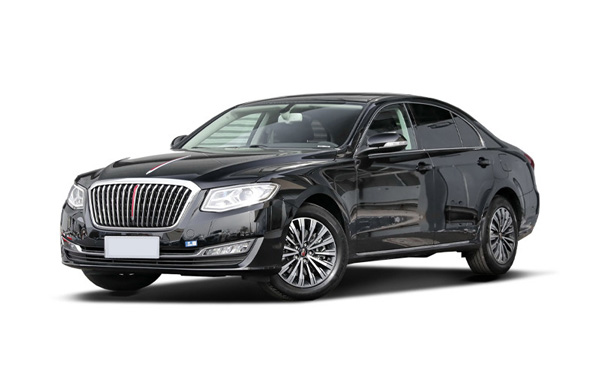•外观
•中控
•座椅
•细节
 本地参考价： 25.28 万元起 厂商指导价：25.28-28.88 万
 车市行情　｜　红旗H7 降万
 最新年款： 级　别：中大型车 结　构：4门5座三厢车 车身颜色： 红色 油　耗：6.8L/100km 排　量：1.8T|2.0T 内饰配色： 红黑 保　修：3年或6万公里 变速箱：6挡手自一体

#### 红旗飘扬 国车荣耀 红旗H7城市驾享汇郑州站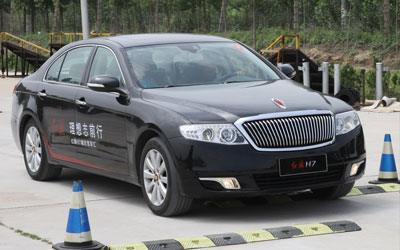### 绕车实拍 外观中控座椅细节全部### 红旗H7 新闻资讯#### 红旗H7轿车选为中国外交车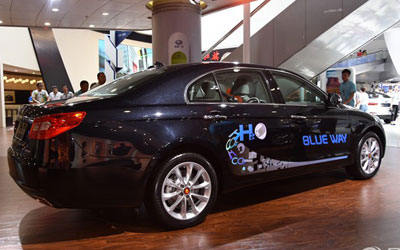#### 一汽红旗H7四驱版震撼发布#### 红旗飘扬 国车荣耀 红旗H7城市驾享汇郑州站

2014年5月24-25日，“红旗H7城市驾享汇”活动在中原绿城-郑州激情绽放。红旗为这座历史文化名城带来了一场非比寻常的“试”看盛宴。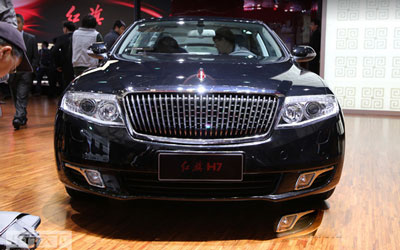#### 一汽红旗H7全系车型导购 豪华型可作首选#### 红旗H7店内现车紧张 暂无优惠#### 试驾红旗H7 3.0L尊贵版 亲民化转身#### 红旗H7对比奥迪A6L 谁主沉浮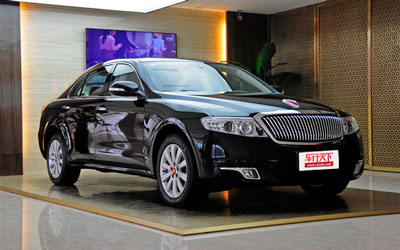#### 送免费常规保养 红旗H7店内少量现车#### 红旗H7正式上市 售29.98-47.98万元

5月30日，一汽红旗旗下全新车型H7正式上市。此次上市的红旗H7包括两种排量共五款车型。售价区间为29.98-47.98万元。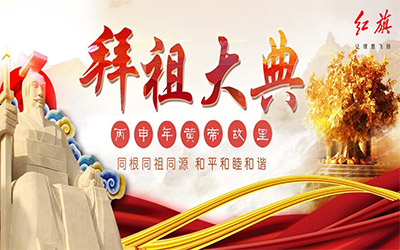#### 大展红旗H7再助2016黄帝故里拜祖大典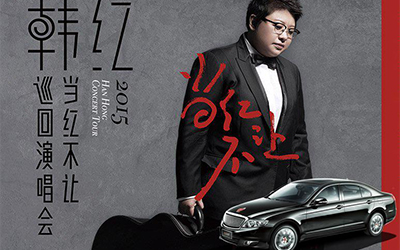#### 郑州大展红旗赞助韩红巡回演唱会

2016年1月1日，韩红当红不让世界巡回演唱会郑州站，即将隆重开启。一场声势浩大的演出，一位天籁歌后的霸气！再加上郑州大展红旗H7的鼎力相助...#### 郑州大展红旗H7精英驾驭礼享完美收官

2015年12月5日，郑州大展红旗店以“理想品悦未来”为主题的红旗H7精英驾驭礼享日活动，作为2015年度最后一场厂家促销团购会完美收官。本次活动...#### 公车改革 首选郑州大展红旗H7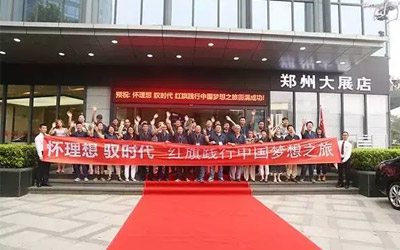#### 郑州大展红旗践行中国梦之旅落幕

8月1日-2日，“怀理想驭时代——红旗践行中国梦想之旅”在河南郑州荣耀启程。22台红旗H7，几十名忠诚用户，12小时深度试驾，700公里行程，品味...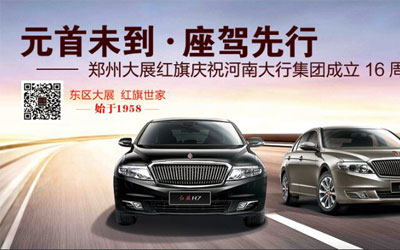#### 红旗H7中原福塔车展招募中

7月26日，由汽车之家主办的福塔车展，红旗H7亲临福塔车展现场，给您带来视觉上的新体验，在享受红旗H7高大上的同时，也为您展示红旗H7在这夏日...#### 郑州大展红旗赞助群象岛2015峰会圆满成功

2015年5月28日，由郑州大展红旗赞助的群象岛2015夏季峰会圆满完成，来自河南各行各业的企业掌门人和经济学人齐聚一堂，共同见证了整个峰会。峰...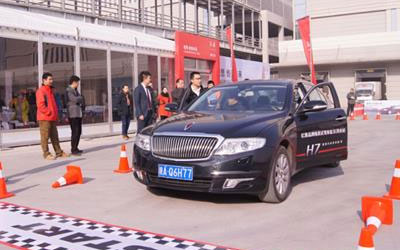### 猜你喜欢

﻿
• 快速找车
• 选择品牌
• 选择品牌
• A  奥迪
• A  阿斯顿·马丁
• A  阿尔法·罗密欧
• B  宝沃
• B  布加迪
• B  巴博斯
• B  保时捷
• B  宾利
• B  奔驰
• B  宝马
• B  本田
• B  别克
• B  标致
• B  比亚迪
• B  宝骏
• B  北汽制造
• B  北汽新能源
• B  北汽幻速
• B  北汽威旺
• B  北京汽车
• B  奔腾
• B  北汽绅宝
• B  北汽昌河
• C  长安欧尚
• C  长安
• C  长安凯程
• C  长城
• D  大众
• D  道奇
• D  DS
• D  东南
• D  东风风神
• D  东风风行
• D  东风小康
• D  东风风度
• D  东风
• F  福特
• F  丰田
• F  菲亚特
• F  法拉利
• F  福田
• F  福迪
• F  福汽启腾
• G  观致
• G  广汽传祺
• G  广汽吉奥
• G  GMC
• H  红旗
• H  汉腾汽车
• H  哈弗
• H  哈飞
• H  海格
• H  海马
• H  华颂
• H  黄海
• H  华泰
• H  恒天
• J  几何汽车
• J  捷达
• J  吉利汽车
• J  捷豹
• J  Jeep
• J  江淮
• J  江铃
• J  金杯
• J  九龙
• J  金旅
• K  凯翼
• K  凯迪拉克
• K  克莱斯勒
• K  科尼塞克
• K  卡威
• K  开瑞
• L  路虎
• L  林肯
• L  劳斯莱斯
• L  兰博基尼
• L  雷克萨斯
• L  铃木
• L  领克
• L  雷诺
• L  理念
• L  力帆
• L  莲花汽车
• L  猎豹
• L  路特斯
• L  陆风
• M  马自达
• M  MG
• M  MINI
• M  玛莎拉蒂
• M  摩根
• M  迈凯轮
• N  纳智捷
• O  欧拉
• O  欧宝
• O  讴歌
• O  欧朗
• Q  奇瑞
• Q  起亚
• Q  启辰
• R  日产
• R  荣威
• R  瑞麒
• S  SERES赛力斯
• S  三菱
• S  斯威汽车
• S  萨博
• S  smart
• S  斯柯达
• S  斯巴鲁
• S  思铭
• S  双龙
• S  上汽大通
• S  双环
• T  特斯拉
• T  腾势
• W  蔚来
• W  沃尔沃
• W  WEY
• W  五菱汽车
• W  五十铃
• W  威兹曼
• W  威麟
• X  现代
• X  雪佛兰
• X  星途
• X  雪铁龙
• X  小鹏汽车
• X  西雅特
• Y  一汽
• Y  英菲尼迪
• Y  英致
• Y  依维柯
• Y  野马汽车
• Y  永源
• Z  众泰
• Z  中华
• Z  中兴
• Z  知豆
• 选择车系
• 选择车系
• 车型对比
• 选择品牌
• 选择品牌
• A  奥迪
• A  阿斯顿·马丁
• A  阿尔法·罗密欧
• B  宝沃
• B  布加迪
• B  巴博斯
• B  保时捷
• B  宾利
• B  奔驰
• B  宝马
• B  本田
• B  别克
• B  标致
• B  比亚迪
• B  宝骏
• B  北汽制造
• B  北汽新能源
• B  北汽幻速
• B  北汽威旺
• B  北京汽车
• B  奔腾
• B  北汽绅宝
• B  北汽昌河
• C  长安欧尚
• C  长安
• C  长安凯程
• C  长城
• D  大众
• D  道奇
• D  DS
• D  东南
• D  东风风神
• D  东风风行
• D  东风小康
• D  东风风度
• D  东风
• F  福特
• F  丰田
• F  菲亚特
• F  法拉利
• F  福田
• F  福迪
• F  福汽启腾
• G  观致
• G  广汽传祺
• G  广汽吉奥
• G  GMC
• H  红旗
• H  汉腾汽车
• H  哈弗
• H  哈飞
• H  海格
• H  海马
• H  华颂
• H  黄海
• H  华泰
• H  恒天
• J  几何汽车
• J  捷达
• J  吉利汽车
• J  捷豹
• J  Jeep
• J  江淮
• J  江铃
• J  金杯
• J  九龙
• J  金旅
• K  凯翼
• K  凯迪拉克
• K  克莱斯勒
• K  科尼塞克
• K  卡威
• K  开瑞
• L  路虎
• L  林肯
• L  劳斯莱斯
• L  兰博基尼
• L  雷克萨斯
• L  铃木
• L  领克
• L  雷诺
• L  理念
• L  力帆
• L  莲花汽车
• L  猎豹
• L  路特斯
• L  陆风
• M  马自达
• M  MG
• M  MINI
• M  玛莎拉蒂
• M  摩根
• M  迈凯轮
• N  纳智捷
• O  欧拉
• O  欧宝
• O  讴歌
• O  欧朗
• Q  奇瑞
• Q  起亚
• Q  启辰
• R  日产
• R  荣威
• R  瑞麒
• S  SERES赛力斯
• S  三菱
• S  斯威汽车
• S  萨博
• S  smart
• S  斯柯达
• S  斯巴鲁
• S  思铭
• S  双龙
• S  上汽大通
• S  双环
• T  特斯拉
• T  腾势
• W  蔚来
• W  沃尔沃
• W  WEY
• W  五菱汽车
• W  五十铃
• W  威兹曼
• W  威麟
• X  现代
• X  雪佛兰
• X  星途
• X  雪铁龙
• X  小鹏汽车
• X  西雅特
• Y  一汽
• Y  英菲尼迪
• Y  英致
• Y  依维柯
• Y  野马汽车
• Y  永源
• Z  众泰
• Z  中华
• Z  中兴
• Z  知豆
• 选择车系
• 选择车系
• 选择车型
• 选择车型
• 意见反馈